# Formula bar in Excel: how to show, hide, expand or collapse

In this short tutorial, you will learn what the Excel formula bar is, how to restore a missing formula bar in different versions of Excel, and how to expand the formula bar so that a long formula can fit into it entirely.

On this blog, we have lots of tutorials discussing various aspects of Excel functions and formulas. But if you are a novice in Microsoft Excel, you might want to learn the basics first, and one of the essentials is the Formula Bar.

## What is a formula bar in Excel?

Excel formula bar is a special toolbar at the top of the Excel worksheet window, labeled with function symbol (fx). You can use it to enter a new formula or copy an existing one.

The formula bar comes very handy when you are dealing with a pretty long formula and you want to view it entirely without overlaying the contents of the neighbor cells.

The formula bar gets activated as soon as you type an equal sign in any cell or click anywhere within the bar.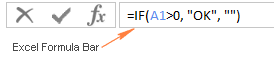## Formula bar missing - how to show formula bar in Excel

Formula bar is very helpful for reviewing and editing formulas in your worksheets. If the formula bar is missing in your Excel, most likely it's because you've accidentally turned off the Formula Bar option on the ribbon. To recover a lost formula bar, perform the following steps.

#### Show formula bar in Excel 2019, Excel 2016, Excel 2013 and Excel 2010

In the modern versions of Excel, you can unhide formula bar by going to the View tab > Show group and selecting the Formula Bar option.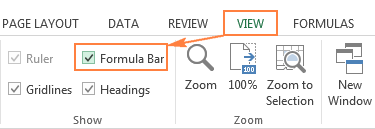#### Show formula bar in Excel 2007

In Excel 2007, the Formula Bar option resides on the View tab > Show/Hide group.

#### Show formula bar in Excel 2003 and XP

In insert the formula bar in the old Excel versions, go to Tools > Options, then switch to the View tab, and select the Formula Bar checkbox under the Show category.

#### Unhide formula bar via Excel Options

An alternative way to restore a lost formula bar in Excel is this:

• Click File (or the Office button in earlier Excel versions).
• Go to Options.
• Click Advanced in the left pane.
• Scroll down to the Display section and select the Show Formula bar option.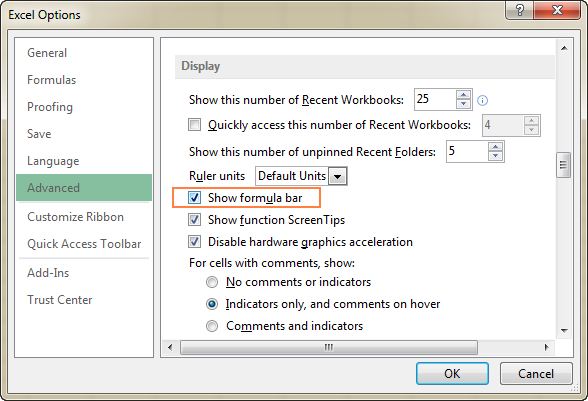## How to hide formula bar in Excel

To maximize workspace in your worksheet, we may want to hide the Excel formula bar. And you can do this by unchecking the Formula bar option in the Excel Options dialog, as demonstrated above, or on the ribbon (View tab > Show group):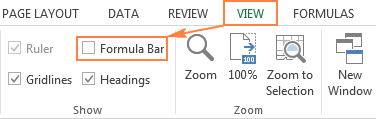## How to expand Excel formula bar

If you are creating an advanced formula that is too long to fit into the default formula bar, you can expand the bar in the following way:

• Hover the mouse near the bottom of the formula bar until you see the up-and-down white arrow.
• Click that arrow and drag down until the bar gets big enough to accommodate the entire formula.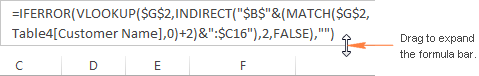#### Formula bar shortcut

Another way to expand the formula bar in Excel is by using the shortcut Ctrl + Shift + U. To restore the default formula bar size, press this shortcut again.

This is how you work with formula bar in Excel. In the next article, we are going to talk about more serious things such as evaluating and debugging Excel formulas. I thank you for reading and hope to see you on our blog next week!